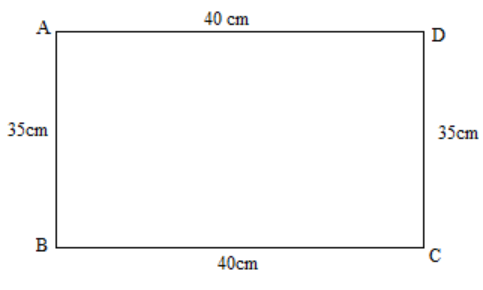Find the perimeter of a rectangle whose:Length = 40cm and breadth = 35cm.Verified
146.4k+ views
Hint: In this question, first we will draw the diagram of the rectangle by indicating the dimensions of the rectangle. We will then see the definition of the perimeter. After this, we will apply the formula to the perimeter of the rectangle to get the required answer.

Complete step-by-step solution:
The diagram for the question is given below:In the question, we have a rectangle with length = 40cm and breadth = 35cm.
Before calculating the perimeter, let us see the definition of the perimeter.
Perimeter:- The perimeter of any geometric figure is the sum of its all sides.
So, for a rectangle, the sum of its all sides is calculated as follow:
Perimeter = $L + L + B +B = 2L +2B =2(L+B)$. ------ (1)
So, the formula for calculating the perimeter of the rectangle is given by equation 1.
Putting the values of length and breadth in equation 1, we get:
Perimeter = $2(L+B) = 2( 40 +35) cm =2 \times 75cm = 150cm$.

Therefore, the required answer is $150cm$.

Note: You should know the definition of the perimeter. No need to remember the formula for the perimeter for different shapes. Once you know the definition, you can calculate the perimeter of any figure by just adding the sides. But you must know the properties of sides of different geometric figures. For example, in a rectangle, the opposite sides are equal and parallel.